A few weeks ago, I was looking through OpenAI's implementation of A2C. The final step in A2C is to generate an action for the agent by sampling from a categorical probability distribution over possible discrete actions. Usually, I've done this with np.random.choice, but OpenAI's implementation was doing it a completely different way. Looking at utils.py, we have:

def sample(logits):
noise = tf.random_uniform(tf.shape(logits))
return tf.argmax(logits - tf.log(-tf.log(noise)), 1)


This sort of makes sense. If you just take the argmax of the logits, you would always just sample the highest-probability action. So instead, you add some noise to the logits to make things more random, and then take the argmax. But why not just use noise? Why -tf.log(-tf.log(noise))?

There's another strange thing. Usually we sample from the distribution created by passing the logits through a softmax function. If we sample using noise on only the logits, does it give the same results?

It turns out this is a clever way of sampling directly from softmax distribution using noise from a special distribution: the Gumbel distribution. Let's explore what this humble distribution is all about.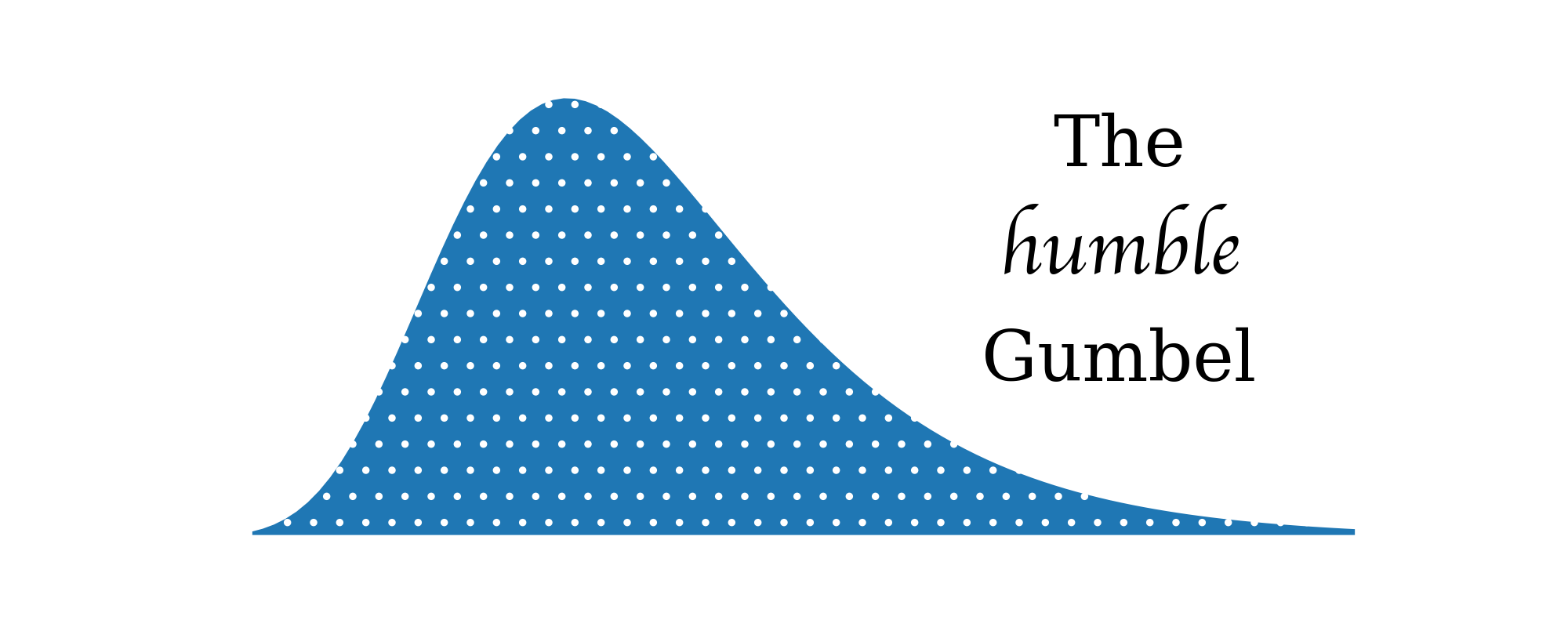(If you'd like to follow along with this notebook interactively, sources can be found at GitHub.)

## The Gumbel distribution¶

The Gumbel distribution is a probability distribution with density function

$$p(x) = \frac{1}{\beta} exp(-z - exp[-z])$$ where $$z = \frac{x - \mu}{\beta}.$$

On its own, the Gumbel distribution is typically used to model the maximum of a set of independent samples. For example, let's say you want to quantify how much ice cream you eat per day. Assume your hunger for ice cream is normally-distributed, with a mean of 5/10. You record your hunger 100 times a day for 10,000 days. (We also assume your hunger is erratic enough that all samples are independent.) You make a note of the maximum hunger you experience every day.

The distribution of daily maximum hunger would then follow a Gumbel distribution.

from scipy.optimize import curve_fit

mean_hunger = 5
samples_per_day = 100
n_days = 10000
samples = np.random.normal(loc=mean_hunger, size=(n_days, samples_per_day))
daily_maxes = np.max(samples, axis=1)

def gumbel_pdf(prob, loc, scale):
z = (prob - loc) / scale
return exp(-z - exp(-z)) / scale

def plot_maxes(daily_maxes):
probs, hungers, _ = hist(daily_maxes, normed=True, bins=100)
xlabel("Hunger")
ylabel("Probability of hunger being daily maximum")

(loc, scale), _ = curve_fit(gumbel_pdf, hungers[:-1], probs)
plot(hungers, gumbel_pdf(hungers, loc, scale))

figure()
plot_maxes(daily_maxes)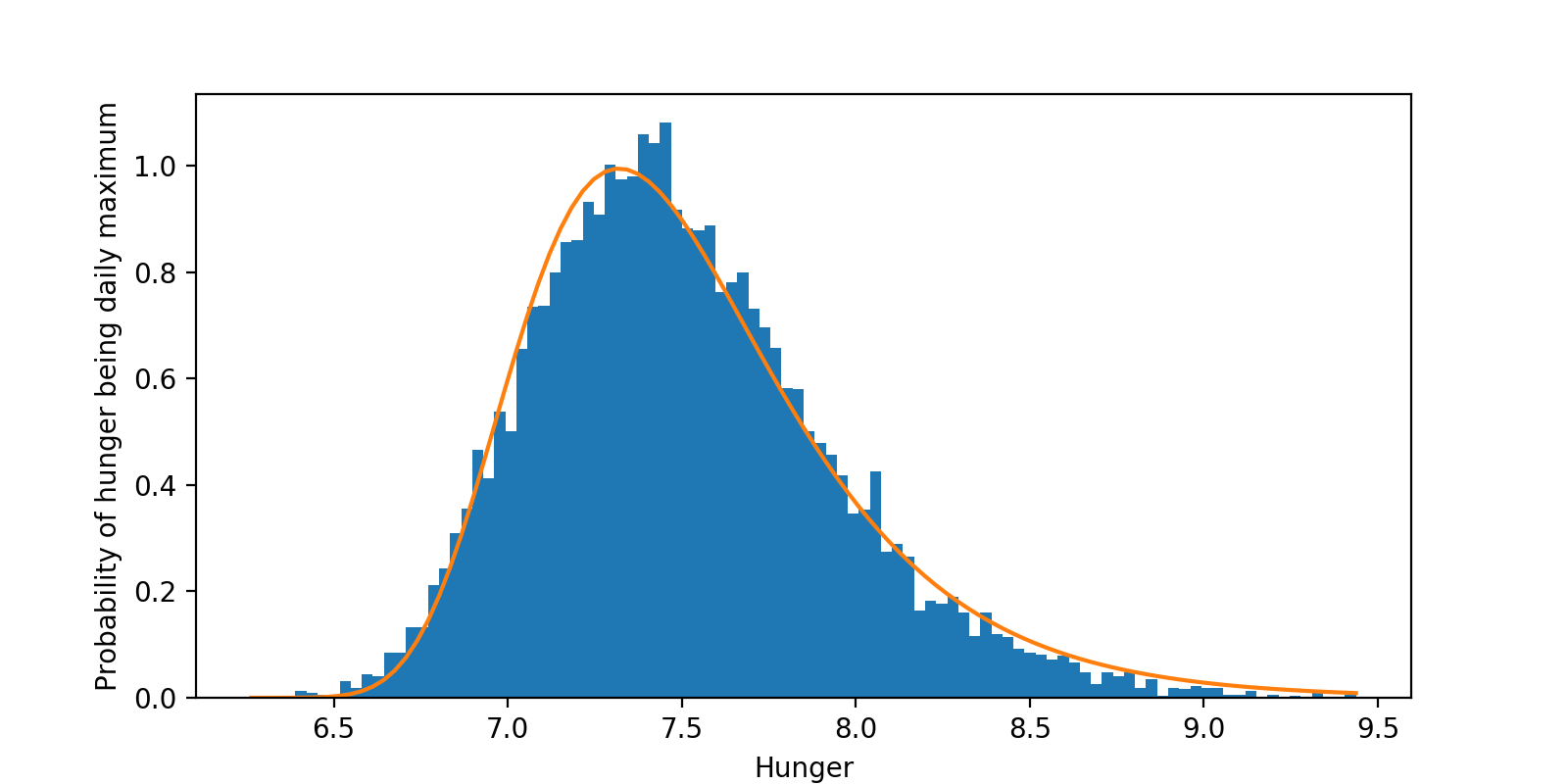From what I understand, the Gumbel distribution should be a good fit when the underlying data is distributed according to either a normal or an exponential distribution. To convince ourselves, let's try again with exponentially-distributed hunger:

most_likely_hunger = 5
samples = np.random.exponential(scale=most_likely_hunger,
size=(n_days, samples_per_day))
daily_maxes = np.max(samples, axis=1)

figure()
plot_maxes(daily_maxes)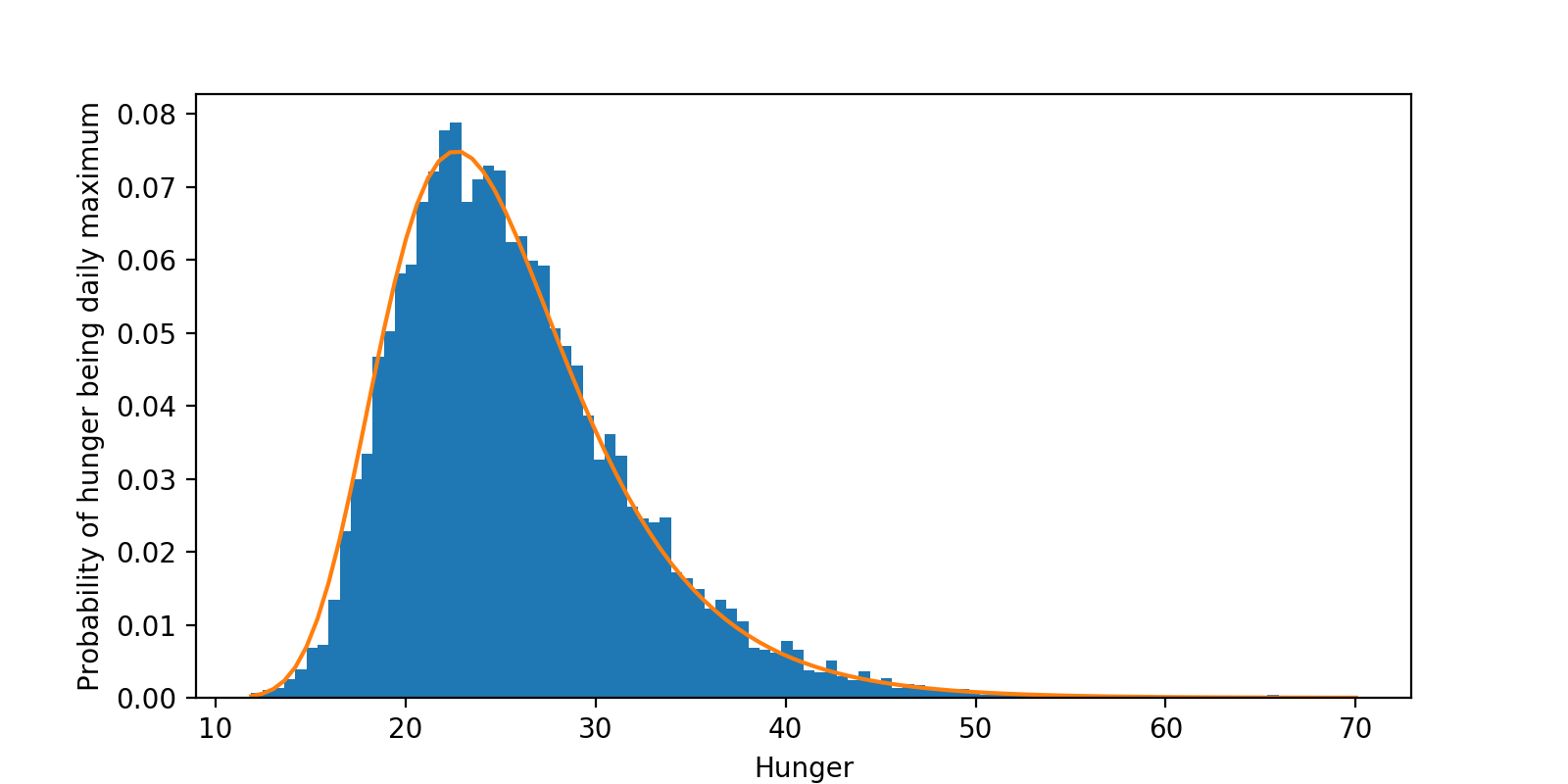Sure enough, the distribution of maximum daily hunger values is still Gumbel-distributed.

Edit: Varun Sudarsanan points out that for exponential distributions, some adjustment has to be done for the sample size. This doesn't show up here because the sample size is only 100, but it becomes more relevant the larger the sample size. Thanks, Varun!

## The Gumbel-max trick¶

What does the Gumbel distribution have to do with sampling from a categorical distribution?

To experiment, let's set up a distribution to work with.

n_cats = 7
cats = np.arange(n_cats)

probs = np.random.randint(low=1, high=20, size=n_cats)
probs = probs / sum(probs)
logits = log(probs)

def plot_probs():
bar(cats, probs)
xlabel("Category")
ylabel("Probability")

figure()
plot_probs()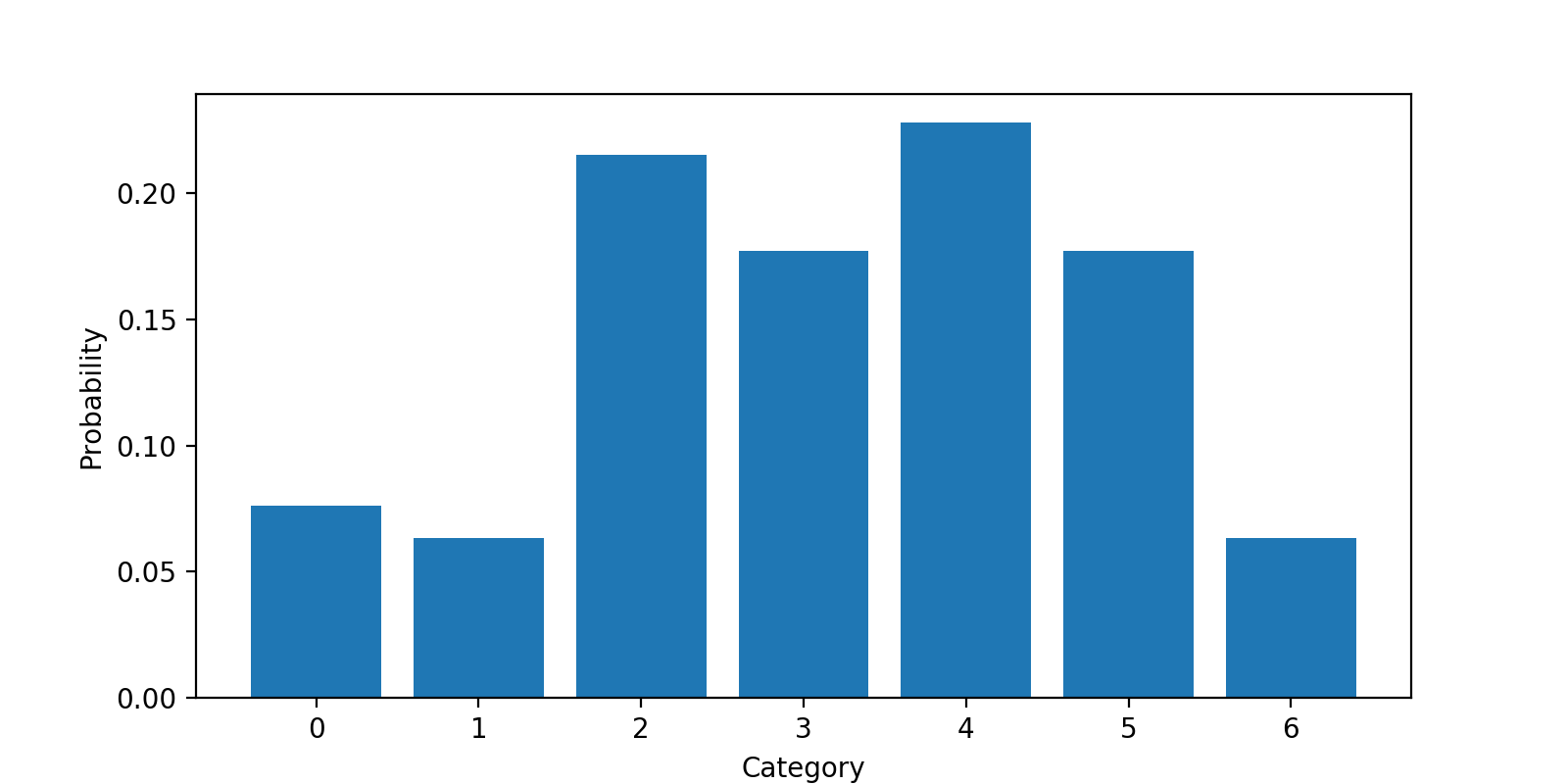As a sanity check, let's try sampling with np.random.choice. Do we get the right probabilities?

n_samples = 1000

def plot_estimated_probs(samples):
n_cats = np.max(samples) + 1
estd_probs, _, _ = hist(samples,
bins=np.arange(n_cats + 1),
align='left',
edgecolor='white',
normed=True)
xlabel("Category")
ylabel("Estimated probability")
return estd_probs

def print_probs(probs):
print(" ".join(["{:.2f}"] * len(probs)).format(*probs))

samples = np.random.choice(cats, p=probs, size=n_samples)

figure()
subplot(1, 2, 1)
plot_probs()
subplot(1, 2, 2)
estd_probs = plot_estimated_probs(samples)
tight_layout()

print("Original probabilities:\t\t", end="")
print_probs(probs)
print("Estimated probabilities:\t", end="")
print_probs(estd_probs)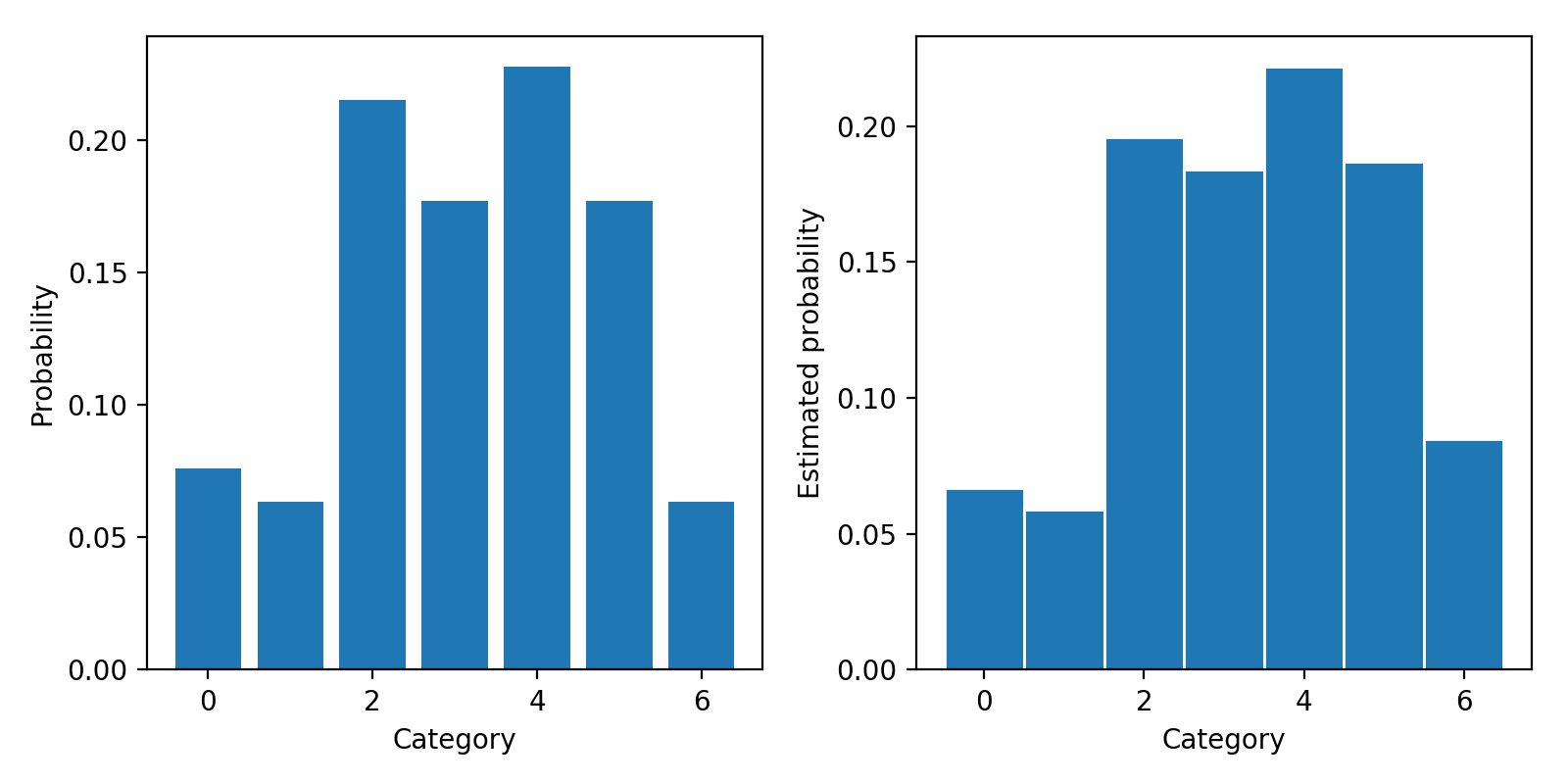Original probabilities:		0.08 0.06 0.22 0.18 0.23 0.18 0.06
Estimated probabilities:	0.07 0.06 0.20 0.18 0.22 0.19 0.09


Looks good.

### Sampling with noise¶

Let's return to OpenAI's interesting way of sampling:

def sample(logits):
noise = tf.random_uniform(tf.shape(logits))
return tf.argmax(logits - tf.log(-tf.log(noise)), 1)


We had some intuition that maybe this works by just using some noise to "shake up" the argmax.

Will any noise do? Let's try a couple of different types.

### Uniform noise¶

def sample(logits):
noise = np.random.uniform(size=len(logits))
sample = np.argmax(logits + noise)
return sample

samples = [sample(logits) for _ in range(n_samples)]

figure()
subplot(1, 2, 1)
plot_probs()
subplot(1, 2, 2)
estd_probs = plot_estimated_probs(samples)
tight_layout()

print("Original probabilities:\t\t", end="")
print_probs(probs)
print("Estimated probabilities:\t", end="")
print_probs(estd_probs)Original probabilities:		0.08 0.06 0.22 0.18 0.23 0.18 0.06
Estimated probabilities:	0.00 0.00 0.34 0.12 0.41 0.13


So uniform noise seems to capture the modes of the distribution, but distorted. It also completely misses out all the other categories.

### Normal noise¶

def sample(logits):
noise = np.random.normal(size=len(logits))
sample = argmax(logits + noise)
return sample

samples = [sample(logits) for _ in range(n_samples)]

figure()
subplot(1, 2, 1)
plot_probs()
subplot(1, 2, 2)
estd_probs = plot_estimated_probs(samples)
tight_layout()

print("Original probabilities:\t\t", end="")
print_probs(probs)
print("Estimated probabilities:\t", end="")
print_probs(estd_probs)